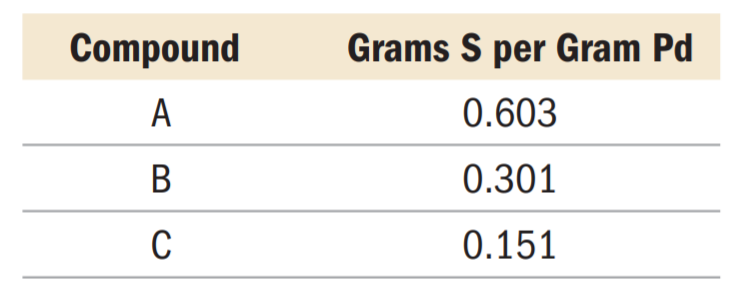×
Get Full Access to Chemistry: A Molecular Approach - 3 Edition - Chapter 2 - Problem 36e
Get Full Access to Chemistry: A Molecular Approach - 3 Edition - Chapter 2 - Problem 36e

×

# Palladium forms three different compounds with sulfur. TheISBN: 9780321809247 1

## Solution for problem 36E Chapter 2

Chemistry: A Molecular Approach | 3rd Edition

• Textbook Solutions
• 2901 Step-by-step solutions solved by professors and subject experts
• Get 24/7 help from StudySoup virtual teaching assistantsChemistry: A Molecular Approach | 3rd Edition

4 5 1 379 Reviews
22
0
Problem 36E

Palladium forms three different compounds with sulfur. The mass of sulfur per gram of palladium in each compound is listed below. Show that these masses are consistent with the law of multiple proportions.Step-by-Step Solution:
Step 1 of 3

Solution 36E Atomic weight of an element is determined by taking ave rage of atomic masses nd respective relative abundance value of its isotopes. The formula for atomic weight is : Now let’s calculate the atomic weight of rubidium(Rb) : Rubidium naturally exists as two isotopes - rubidium-85 and rubidium-87. Given, Atomic mass of Rubidium-85 = 84.9118 amu with abundance of 7 2.15% Atomic mass of Rubidium-87 = 86.9092 amu with abundance of 27.85%. Now using the formula we calculate the atomic weight: =(84.9118 amu)(0.7215)+ (86.9092 amu)(0.2785) [abundance is written in decimals] = 61.26 amu + 24.20 amu = 85.46 amu. Hence, the atomic weight of the rubidium is 85.46 amu

Step 2 of 3

Step 3 of 3

##### ISBN: 9780321809247

This textbook survival guide was created for the textbook: Chemistry: A Molecular Approach, edition: 3. This full solution covers the following key subjects: atomic, rubidium, abundance, AMU, mass. This expansive textbook survival guide covers 82 chapters, and 9454 solutions. The answer to “?Palladium forms three different compounds with sulfur. The mass of sulfur per gram of palladium in each compound is listed below. Show that these masses are consistent with the law of multiple proportions.” is broken down into a number of easy to follow steps, and 33 words. Chemistry: A Molecular Approach was written by and is associated to the ISBN: 9780321809247. The full step-by-step solution to problem: 36E from chapter: 2 was answered by , our top Chemistry solution expert on 02/22/17, 04:35PM. Since the solution to 36E from 2 chapter was answered, more than 466 students have viewed the full step-by-step answer.

Unlock Textbook Solution